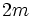# Dihedral and dicyclic groups are isoclinic

## Statement

Suppose$m \ge 2$ is an integer. Then, the following two groups are isoclinic groups:

1. The dicyclic group of degree$m$ and order$4m$.
2. The dihedral group of degree$2m$ and order$4m$.

Further, if$m$ is odd, the nboth of these are also isoclinic to the dihedral group of degree$m$ and order$2m$.

## Particular cases$m$$2m$$4m$ dicyclic group of degree$m$, order$4m$ dihedral group of degree$2m$, order$4m$ If$m$ is odd, dihedral group of degree$m$, order$2m$
2 4 8 quaternion group dihedral group:D8 --
3 6 12 dicyclic group:Dic12 dihedral group:D12 symmetric group:S3
4 8 16 generalized quaternion group:Q16 dihedral group:D16 --
5 10 20 dicyclic group:Dic20 dihedral group:D20 dihedral group:D10